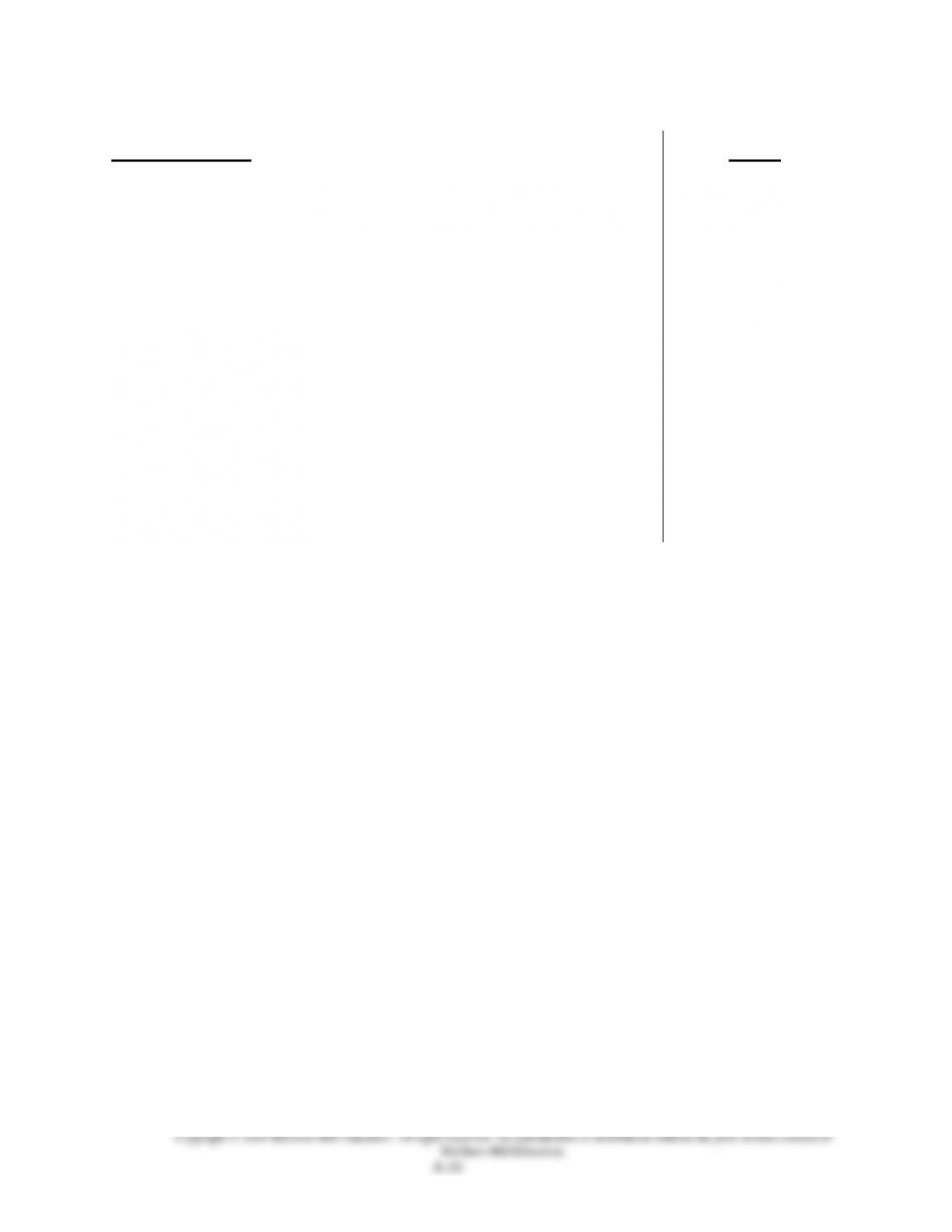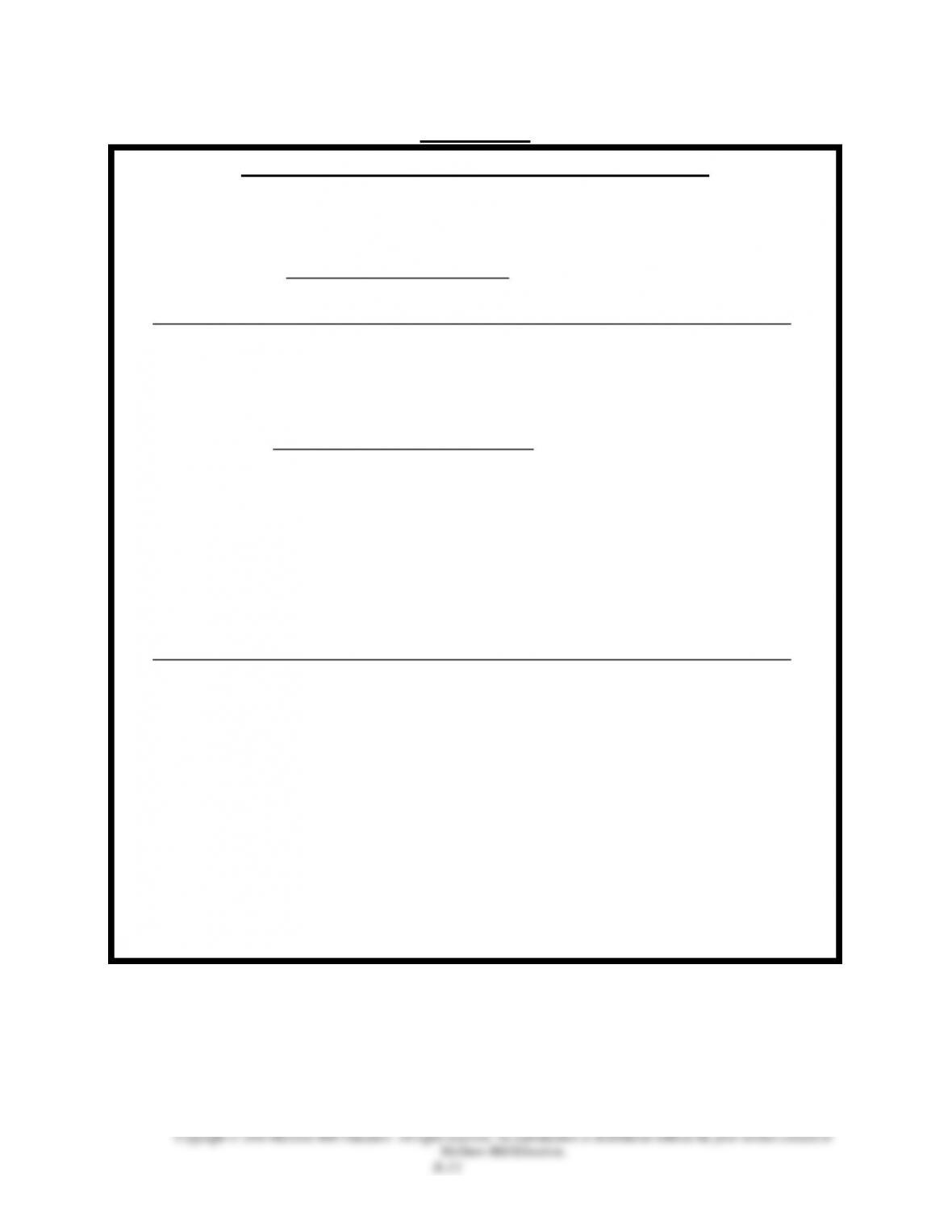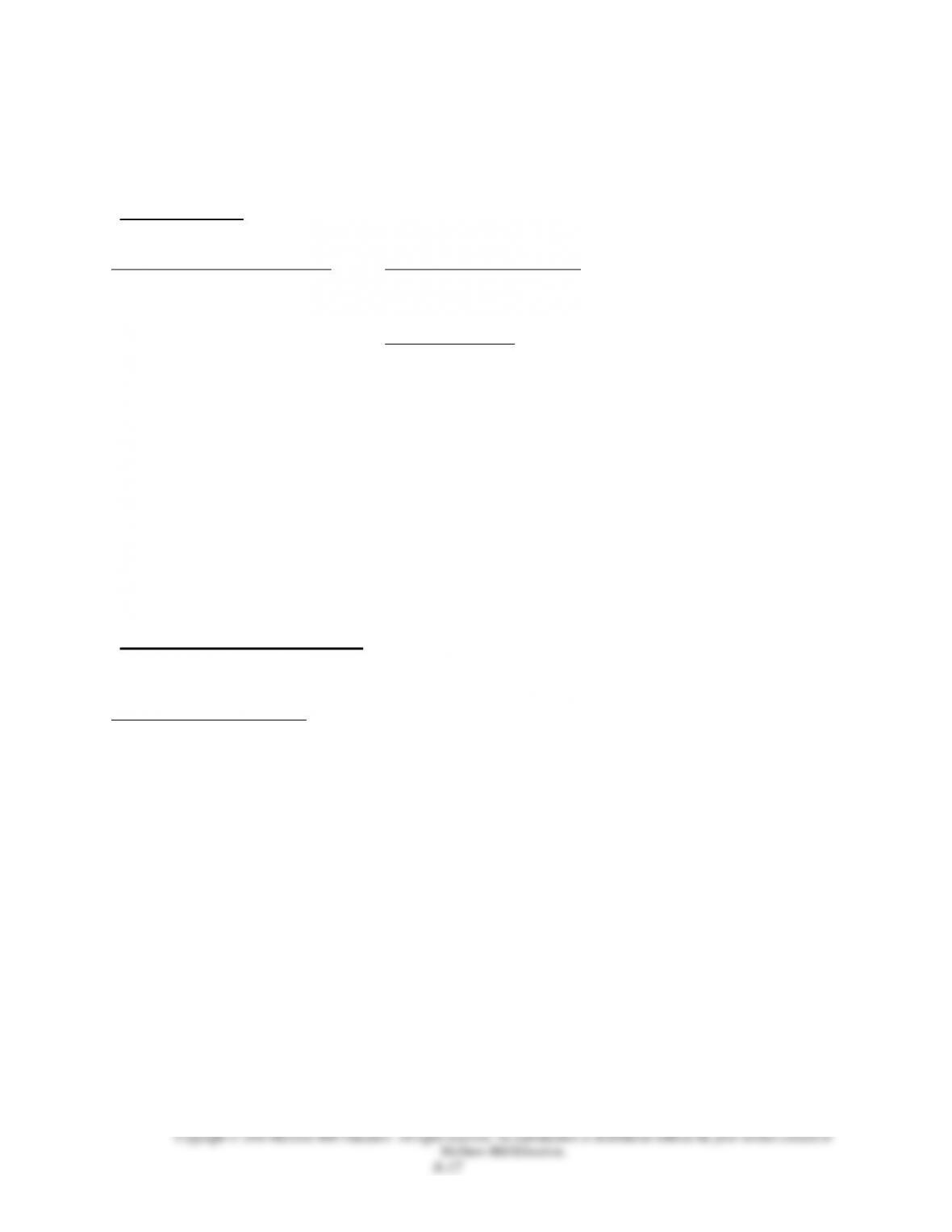Type
Solution Manual
Book Title
Financial Accounting Fundamentals 5th Edition
ISBN 13
978-0078025754

### 978-0078025754 Chapter 8 Lecture Note Part 2

March 26, 2020Chapter Outline
Notes
B. Accounting for Intangiblessimilarities and differences are
consistent with accounting for plant assets however the
requirements for recording increases in value of intangibles is very
restrictive and rare.
VII. Decision AnalysisTotal Asset Turnover
A. Measure of company’s efficiency using assets to generate sales.
B. Calculated by dividing net sales by average total assets.
VIII. Exchanging Plant Assets Appendix 8A
A. Accounting for the exchange depends on whether the transaction
has commercial substance. Commercial substance exists if the
company’s future cash flows change as a result of the transaction.
B. If commercial substance exists, a gain or loss is recorded based on
the difference between the book value of the assets given up and
the market value of the assets received.
C. If exchange lacks commercial substance, no gain or loss is
recorded, and the asset received is recorded based on the book
value of the assets given up.VISUAL #8-1
FORMULAS FOR DEPRECIATION METHODS
1. STRAIGHT LINE
FHC* - Estimated salvage Annual
Estimated useful life Depreciation
*Full Historical Cost
2. UNITS OF PRODUCTION
(Depreciable)
a) FHC - Estimated salvage Cost per
Predicted units of production Unit
b) CPU* x units produced Depreciation
in period for PERIOD
(In last year, depreciate to estimated salvage value; never
depreciate below this amount.)
**Cost Per Unit
3. DOUBLE-DECLINING BALANCE
Book Value (beginning of year) x RATE* = Depreciation (for that year)
*RATE The rate used is constant and it is twice what the
straight line rate would have been for this asset.
(In the last year, depreciate to estimated salvage value; never
depreciate below this amount.)
=
=
=Alternate Demonstration Problem
Chapter 8
A new machine costs \$120,000, has an estimated useful life of five years
and an estimated salvage value of \$15,000 at the end of that time. It is
expected that the machine can produce 210,000 widgets during its useful
life.
The New Times Company purchases this machine on January 1, 2013, and
uses it for exactly three years. During these years the annual production of
widgets has been 80,000, 50,000, and 30,000 units, respectively. On
January 1, 2016, the machine is sold for \$45,000.
Required:
1. Calculate the depreciation expense for each of the first three years
using:
a. Straight-line
b. Units-of-production
c. Double-declining-balance
2. Prepare the proper journal entry for the sale of the machine under the
three different depreciation methods.Solution: Alternate Demonstration Problem
Chapter 8
1a. Straight-line
The depreciation expense each year is equal to cost minus salvage
value divided by useful life. In this example the cost is \$120,000, the
salvage value is \$15,000, and the useful life is 5 years. Therefore,
D
(120,000 - 15,000) / 5
21,000 each year
1b. Units-of-production
The depreciation expense each year is equal to a rate [(cost minus
salvage) divided by total production] multiplied by the actual number
of units produced that year. In this example the rate would be \$0.50
per widget, (120,000 - 15,000) / 210,000, and the depreciation expense
for each of the first three years would be:
D1
.50
x
80,000
40,000
D2
.50
x
50,000
25,000
D3
.50
x
30,000
15,000
1c. Double-declining balance
The depreciation expense each year is equal to a rate (twice the
straight-line rate divided by useful life) multiplied by the asset’s net
book value (cost minus accumulated depreciation) at the beginning
of the year. In this example the rate would be 2/5, or 40%, and the
depreciation expense for each of the first three years would be:
D1
.40
x
120,000
=
48,000
D2
.40
x
72,000
=
28,800
D3
.40
x
43,200
=
17,2802. The journal entry for the sale of the asset will have the same general
form regardless of the method of depreciation adopted, except that
whether there is a gain or a loss on the sale may change according to
the depreciation method used. The gain or loss on disposal of the asset
is determined by comparing the sale price, in this case \$45,000, with the
net book value of the asset at the time of the sale.
Straight-line
Cash .........................................
45,000
Accumulated depreciation .....
63,000
Loss on sale of machine ........
12,000*
Machine ..............................
120,000
* Book value of 57,000 less sale price \$45,000 equals loss of \$12,000
Units-of-production
Cash .........................................
45,000
Accumulated depreciation .....
80,000
Machine ..............................
120,000
Gain on sale of machine ...
5,000
* Sale price \$45,000 less book value of \$40,000 equals gain of \$5,000
Double-declining balance
Cash .........................................
45,000
Accumulated depreciation .....
94,080
Machine ..............................
120,000
Gain on sale of machine ...
19,080
*Sale price \$45,000 less book value of \$25,920 equals gain of \$19,080Alternate Demonstration Problem #2
Chapter 8
A new van costs \$25,000, has an estimated useful life of five years and an
estimated salvage value of \$5,000 at the end of that time. It is expected that
the van will be driven 100,000 miles during its useful or service life.
The Nation Express Company purchases this van on April 1, 2014. During
2014 the van is driven 13,000 miles and during 2015 it was driven 21,000
miles.
Required:
1. Calculate the depreciation expense for 2014 and 2015 using:
a. Straight-line
b. Double-declining-balance
c. Units-of-productionSolution: Alternate Demonstration Problem #2
Chapter 8
Straight Line
Cost - Salvage value
=
25000 - 5000
useful life
5 years
20,000
5
\$4,000
per year
2014: 4,000 x 9/12 = 3,000
2015: 4,000
Double declining balance
2
x
book
value
=
Depreciation expense
life
2014: 2/5
x
25,000
=
10,000
x
9/12 =
7,500
2015: 2/5
17,500
7,000
x
12/12 =
7,000Units of Production
cost - salvage
=
depreciation rate per mile
total miles
Rate
x
actual
mileage
=
Depreciation
expense
25,000 - 5,000
=
\$ 0.20
per mile
(rate)
100,000 miles
rate
x
actual
mileage
=
Expense
2014:
\$ 0.20
x
13,000
=
\$ 2,600
2015:
\$ 0.20
x
21,000
=
\$ 4,200